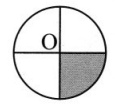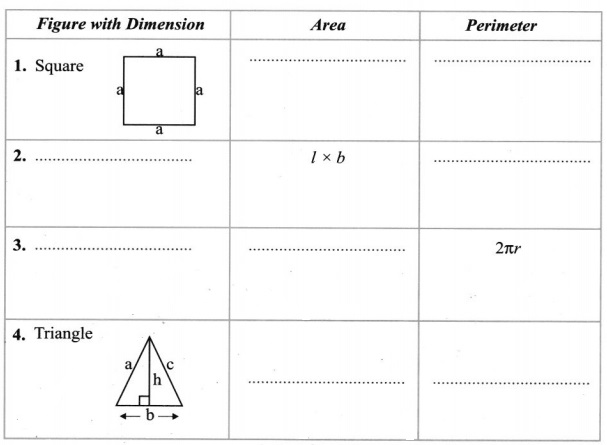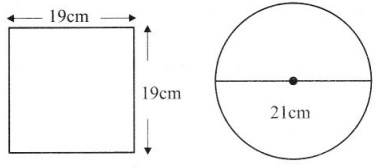# Grade 7 Perimeter and Area Worksheets

## Grade 7 Maths Perimeter and Area Multiple Choice Questions (MCQs)

1. The area of a rectangular sheet is 500 cm2. If the length of the sheet is 25 cm, what is its width?
(a) 20 cm
(b) 17 cm
(c) 30 cm
(d) 25 cm

2. Which figure encloses more area: a square of side 2 cm; a rectangle of side 3 cm & 2 cm; An equilateral triangle of side 4 cm?
(a) rectangle
(b) square
(c) triangle
(d) same of rectangle & square

3. The area of parallelogram is:
(a) base + height
(b) base × height
(c) base × base
(d) height × height

4. Which of the following has the formula: Base × Height
(a) area of parallelogram
(c) area of triangle
(d) area of trapezium

5. If the area of the triangle is 36 cm2 and the height is 3 cm, the base of the triangle will be:
(a) 12 cm
(b) 39cm
(c) 108 cm
(d) 24 cm

6. The perimeter of square of side 2.55 m is:
(a) 10.2 m
(b) 10.2 m2
(c) 6.25 m2
(d) 6.25 m

7. Area of triangle whose base is 15 cm and corresponding altitude is 6 cm will be:
(a) 45 cm2
(b) 90 cm2
(c) 45 cm
(d) 90 cm

8. The circumference of circle whose diameter is 14 cm will be:
(a) 44 cm
(b) 88 cm
(c) 44 cm2
(d) 88 cm2

9. If the area of circle is 44 cm2, the area of shaded portion will be:
(a) 11 cm2
(b) 11 cm
(c) 22 cm2
(d) 22 cm210. The diameter of a circle is:
(a) r2
(b) 2r
(c) 2πr3
(d) πr2

11. 1 m2 = ………………
(a) 100 cm2
(b) 1000 cm2
(c) 10000 m2
(d) 10000 cm2

12. The value of constant π is:
(a) 31.4
(b)$\frac{22}{7}$
(c)$\frac{7}{22}$
(d) 314

13. The difference between the circumference and radius of a circle is 37 cm. The area of the circle is:
(a) 111 cm2
(b) 184 cm2
(c) 154 cm2
(d) 259 cm2

14. On decreasing the radius of the circle by 30%, its area is decreased by:
(a) 30%
(b) 60%
(c) 45%
(d) none of these

15. The diameter of a wheel is 40 cm. How many revolutions will it make on covering 176 m?
(a) 140
(b) 150
(c) 160
(d) 166

16. Find the area of a circle whose circumference is 52.8 cm.
(a) 221.76 cm2
(b) 220.76 cm2
(c) 200.76 cm2
(d) none of these

17. If the perimeter of a semicircular protractor is 36 cm, find the diameter.
(a) 14 cm
(b) 16 cm
(c) 18 cm
(d) 12 cm

18. A wire is in the shape of a square of side 10 cm. If the wire is rebent into a rectangle of length 12 cm, find its breadth.
(a) 12 cm
(b) 7 cm
(c) 8 cm
(d) 9 cm

19. Find the area of the shaded region in the adjacent figure, take π = 3.14
(a) 75 cm2
(b) 72 cm2
(c) 70 cm2
(d) none of these20. The area of a square and a rectangle are equal. If the side of the square is 40 cm and the breadth of the rectangle is 25 cm, find the length of the rectangle.
(a) 60 m
(b) 62 m
(c) 64 m
(d) 68 m

### Grade 7 Maths Perimeter and Area Fill In The Blanks

1. 10000 m2 = ………………. hectare.
2. 1 m2 = ……………. cm2.
3. Perimeter of a regular polygon = length of one side × ………………. .
4. The distance around a circle is its ……………… .
5. If a wire in the shape of the square is rebent into a rectangle, then the …………….. of both shapes remain same, but …………………. may vary.

### Grade 7 Maths Perimeter and Area True(T) or False(F)

1. All the triangles that are equal in area are congruent.
2. All congruent triangles are equal in area.
3. Ratio of the circumference and the diameter of a circle is more than 3.
4.figure (b) has greater perimeter than figure (a)
5. If the area of rectangle increases from 2 cm2 to 4 cm2, then perimeter will remains same.

### Grade 7 Maths Perimeter and Area Very Short Answer Type Questions

Complete the following Table:1. Perimeter of a square park is 2000m. Find its area.
2. A rectangular plot is 75 metre long and 60 metre broad. It has a path of width 2 metre all around it inside. Find the area of the path.
3. From a circular sheet of radius 4cm, a circle of radius 3cm is removed. Find the area of the remaining sheet (Take π = 3.14).
4. The ratio of radii of two circles is 4:5. Find the ratio of their areas.